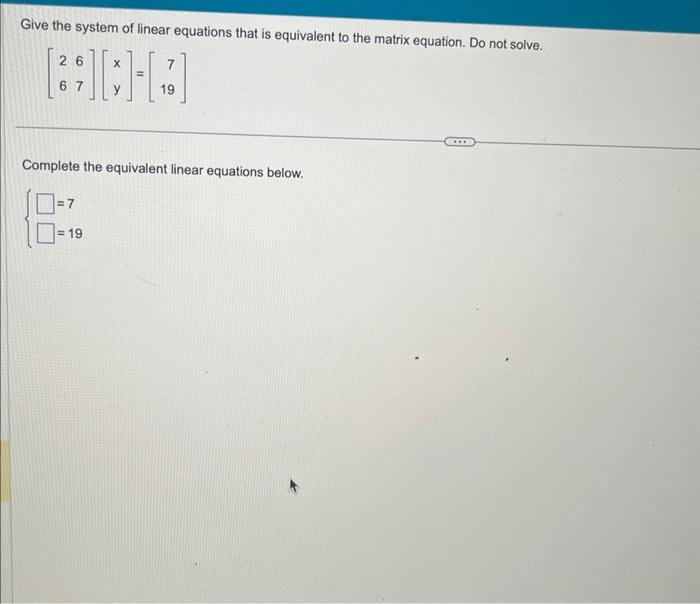Home / Expert Answers / Other Math / 14-give-the-system-of-linear-equations-that-is-equivalent-to-the-matrix-equation-do-not-solve-pa282

# (Solved): 14 Give the system of linear equations that is equivalent to the matrix equation. Do not solve. $\ ... 14Give the system of linear equations that is equivalent to the matrix equation. Do not solve. \[ \left[\begin{array}{ll} 2 & 6 \\ 6 & 7 \end{array}\right]\left[\begin{array}{l} x \\ y \end{array}\right]=\left[\begin{array}{r} 7 \\ 19 \end{array}\right]$ Complete the equivalent linear equations below. $\left\{\begin{array}{l} =7 \\ =19 \end{array}\right.$

We have an Answer from Expert